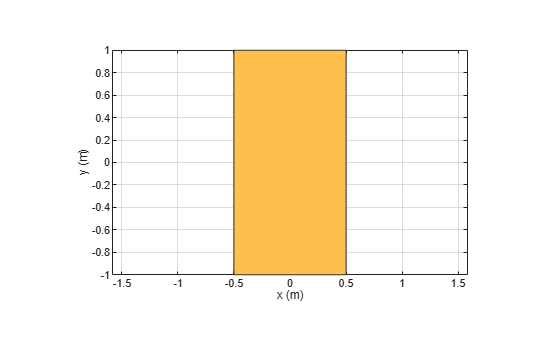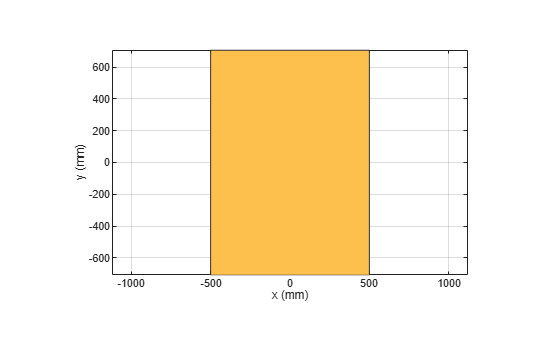# rotateX

Rotate shape about x-axis by angle

## Syntax

``rotateX(shape,angle)``
``c = rotateX(shape,angle)``

## Description

example

````rotateX(shape,angle)` rotates the shape about x-axis by an angle and plots the resultant shape.```
````c = rotateX(shape,angle)` rotates the shape about x-axis by an angle and returns a shape object.```

## Examples

collapse all

Create a rectangle shape.

```r = antenna.Rectangle; show(r) axis equal```Rotate the rectangle at 45 degrees about the x-axis.

`r1 = rotateX(r,45)`
```r1 = Rectangle with properties: Name: 'myrectangle' Center: [0 0] Length: 1 Width: 2 NumPoints: 2 ```
```show(r1) axis equal```## Input Arguments

collapse all

2-D and 3-D geometric shapes created using shape objects in Custom 2-D and 3-D Antenna catalog.

Example: `antenna.Rectangle`

Example: `shape.Polygon`

Example: `shape.Box`

Angle of rotation, specified as a scalar in degrees.

Example: `45`

Data Types: `double`

## Output Arguments

collapse all

Resultant shape obtained after the rotation operation on the input shape, returned as a shape object.

Example: `antenna.Rectangle`

Example: `shape.Polygon`

Example: `shape.Box`

## Version History

Introduced in R2017a